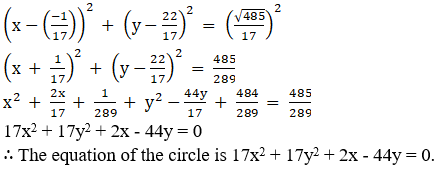# RD Sharma Solutions for Class 11 Maths Exercise 24.1 Chapter 24 - The Circle

Here, in this exercise, we mainly deal with finding the equation of a circle when its centre and radius are known, on finding the centre and radius of a given circle. RD Sharma Class 11 Maths Solutions are provided here in order to help students secure good marks in the board exams. The solutions are designed by our expert faculty team in a step by step manner to help students understand the concepts clearly. Students are advised to practice on a daily basis, which yields good results in the board exams. The pdf of RD Sharma Solutions are provided in the links given below, which can be downloaded easily and can be used for future reference as well.

## Download the pdf of RD Sharma Solutions for Class 11 Maths Exercise 24.1 Chapter 24 – The Circle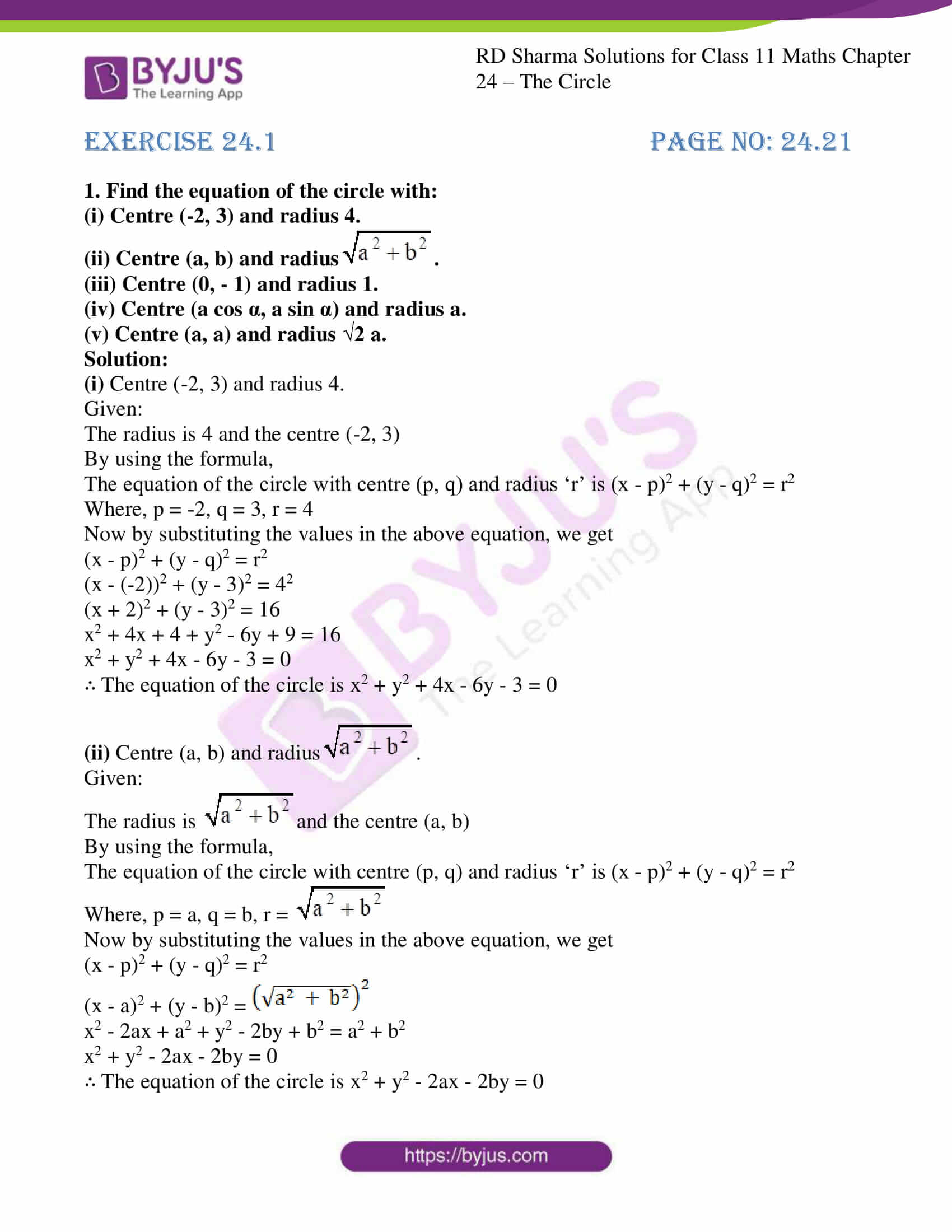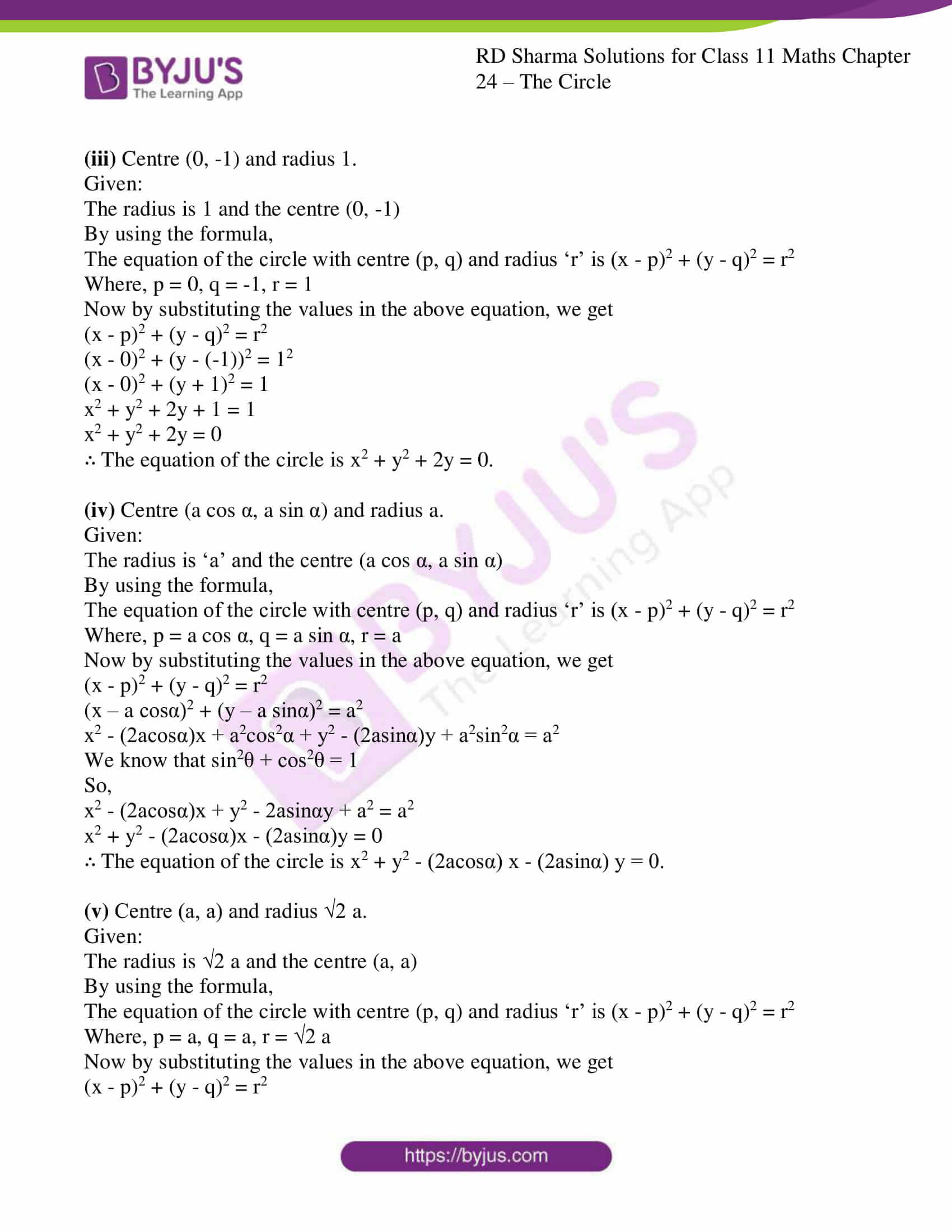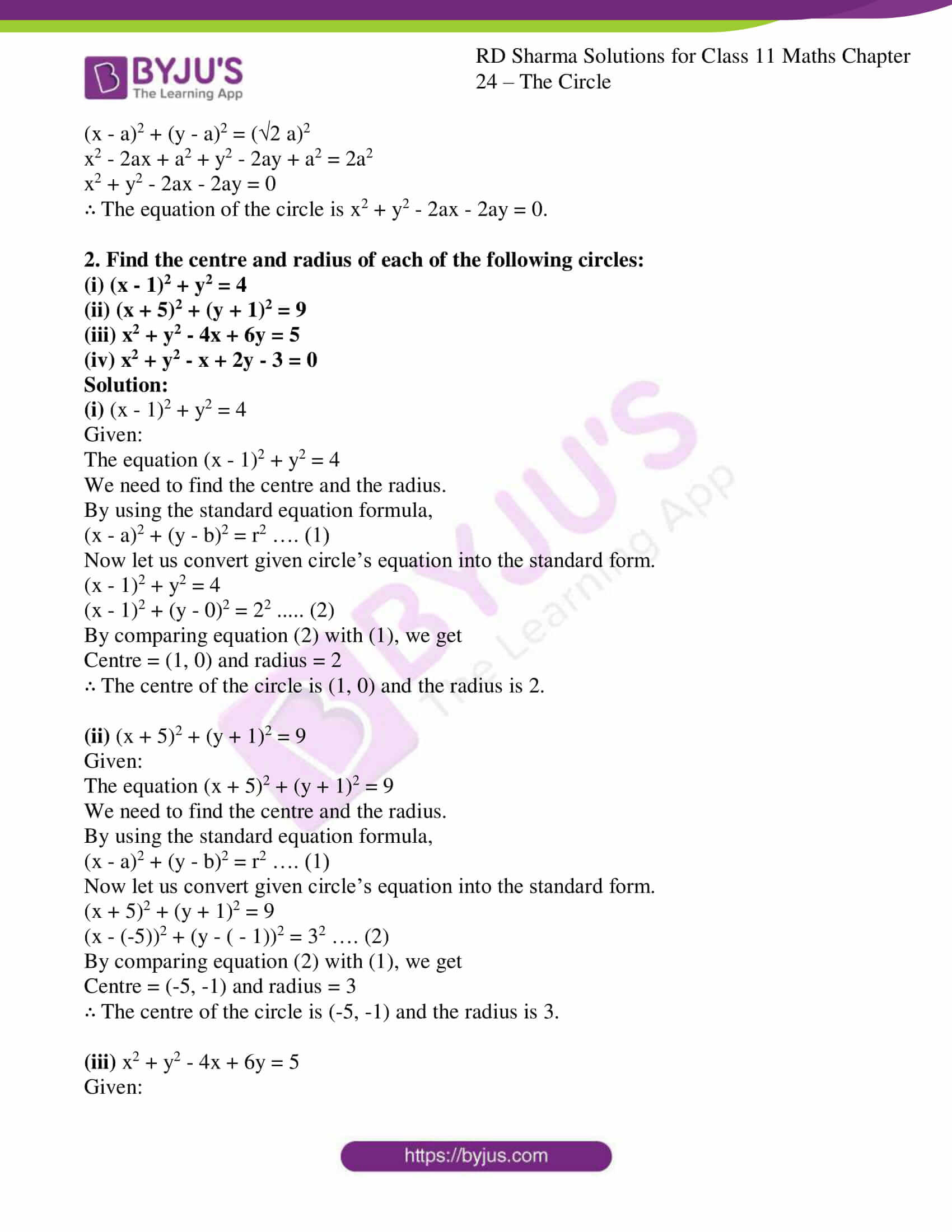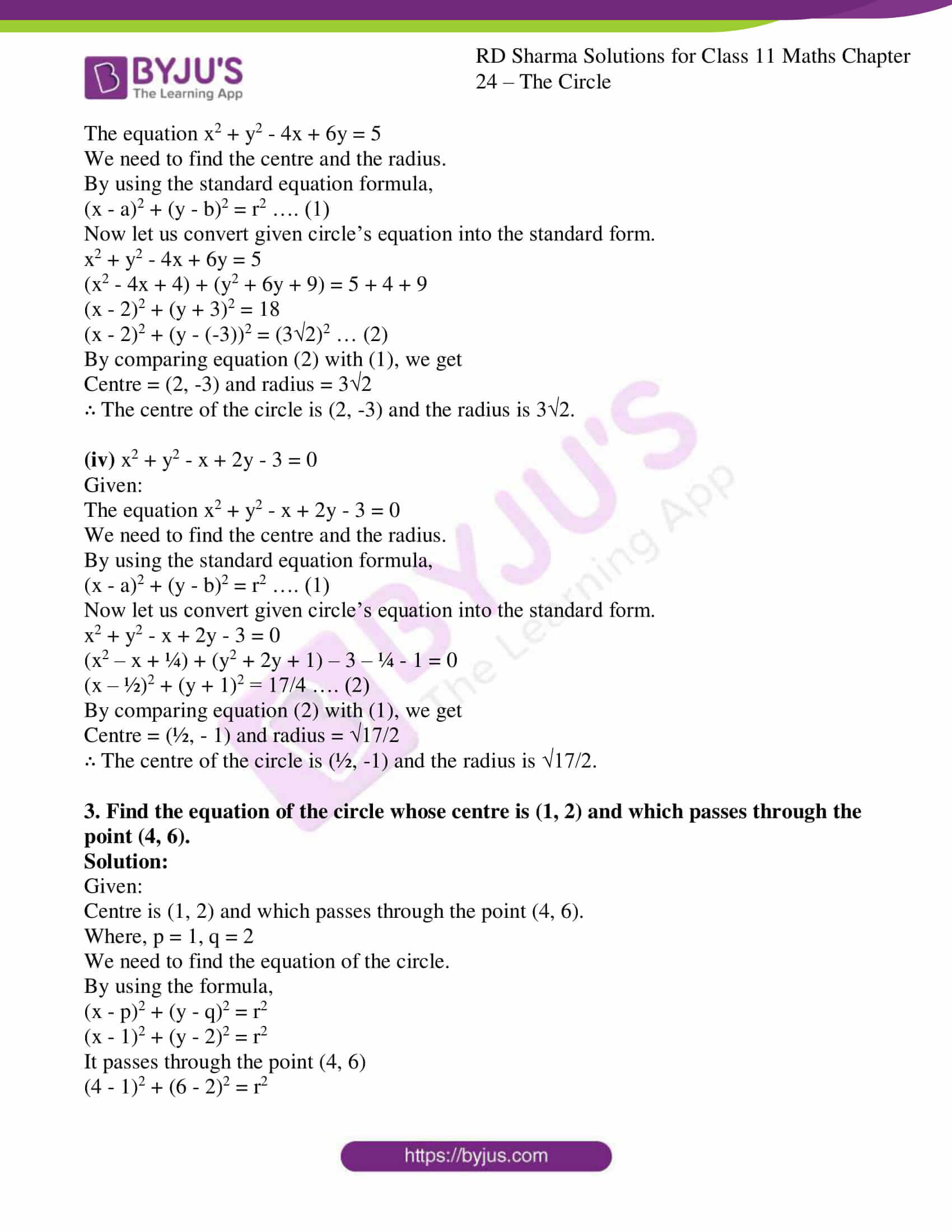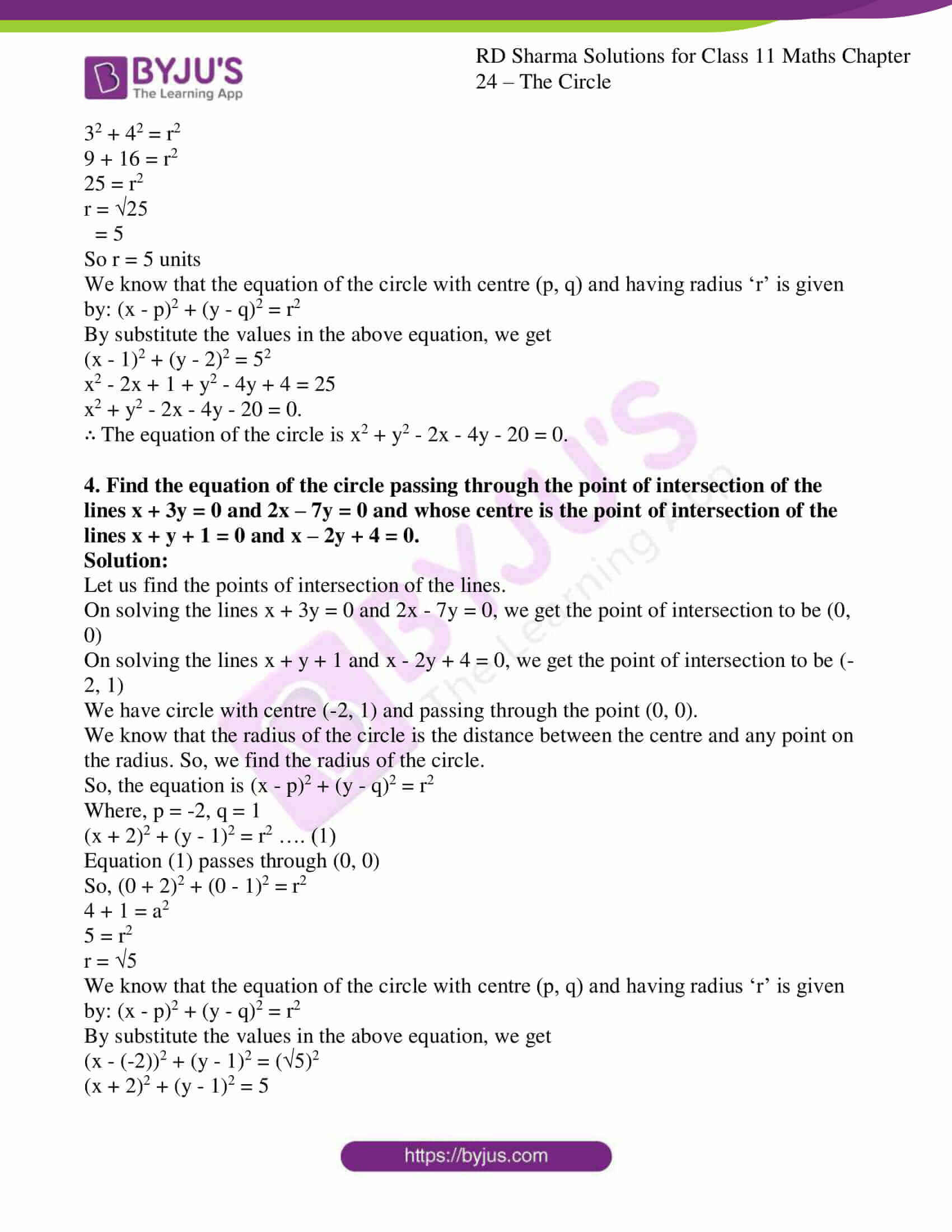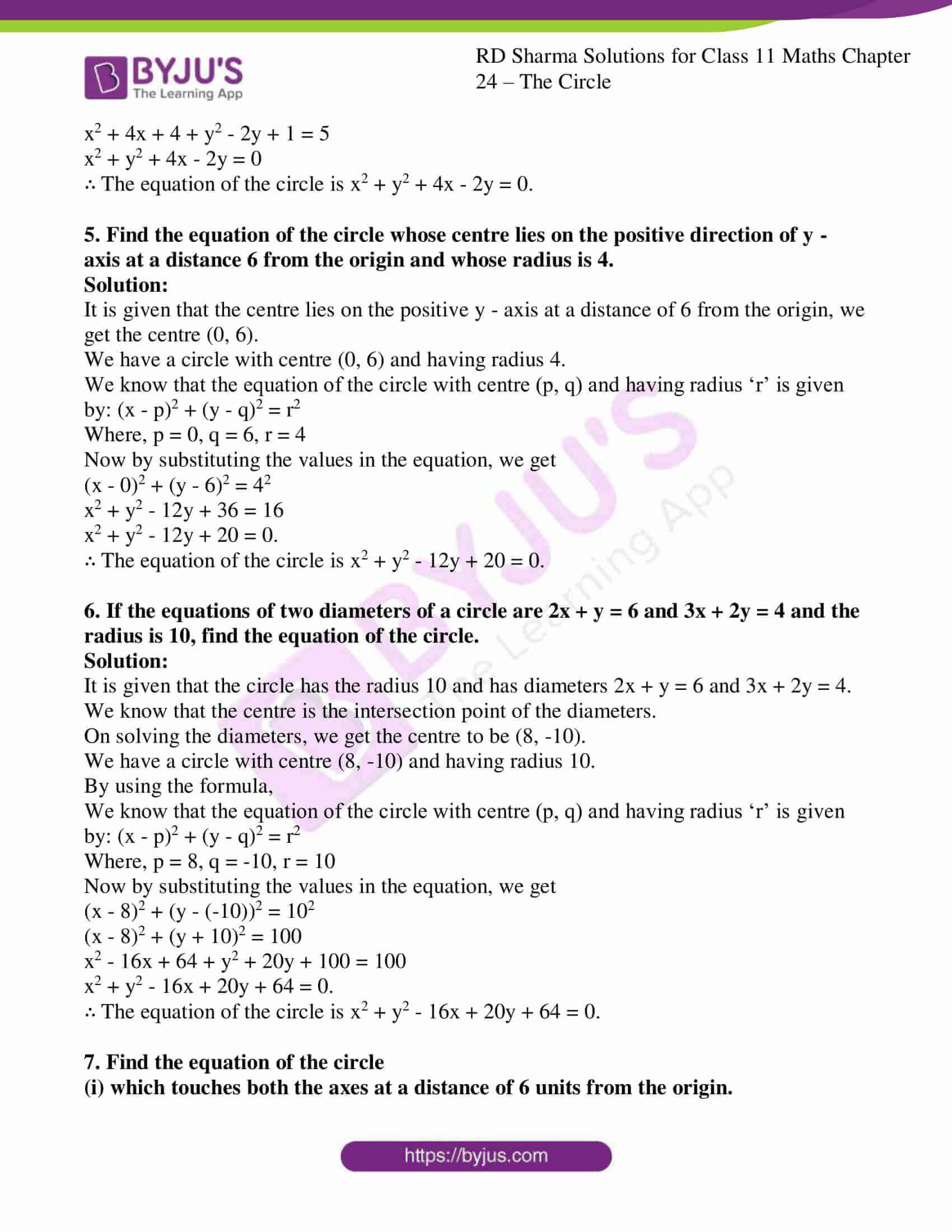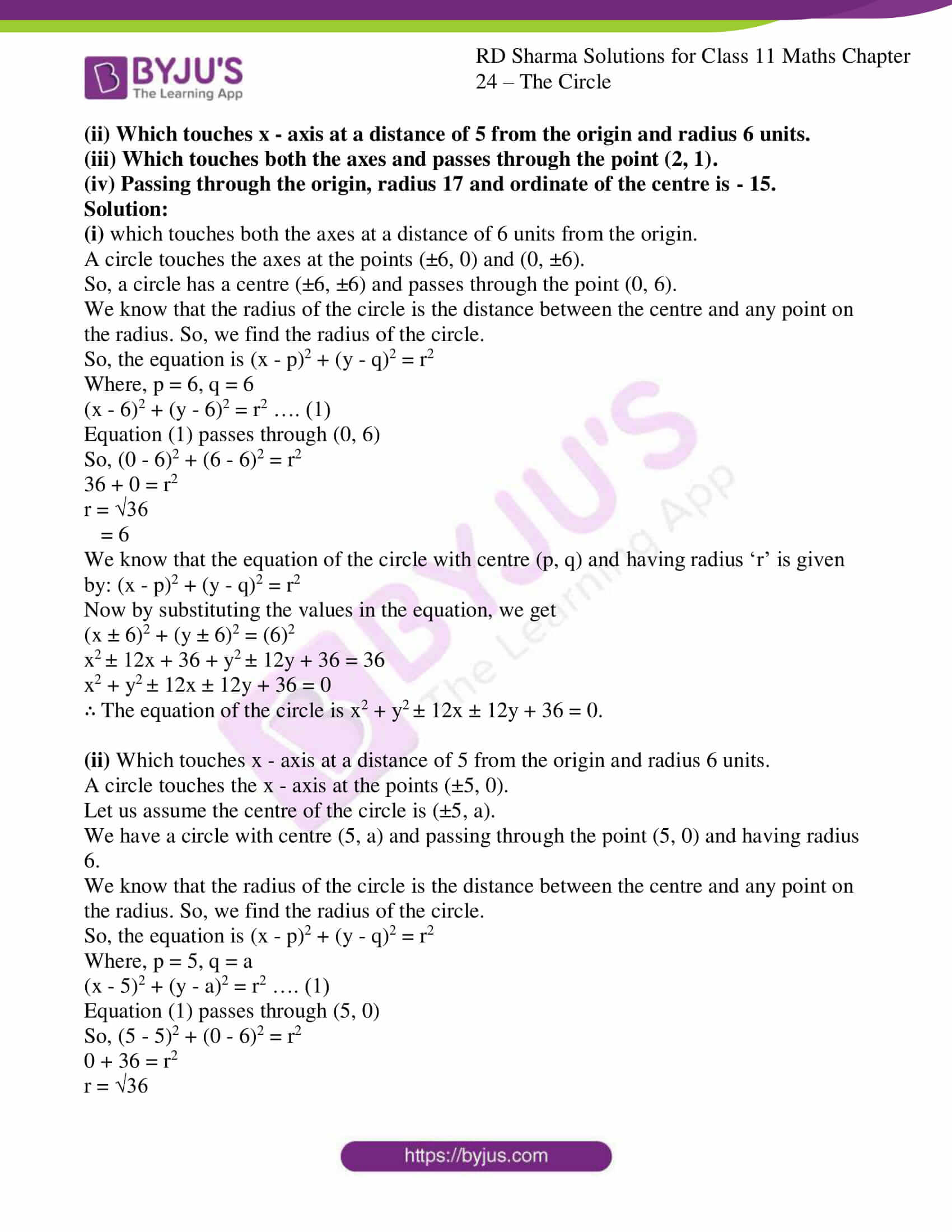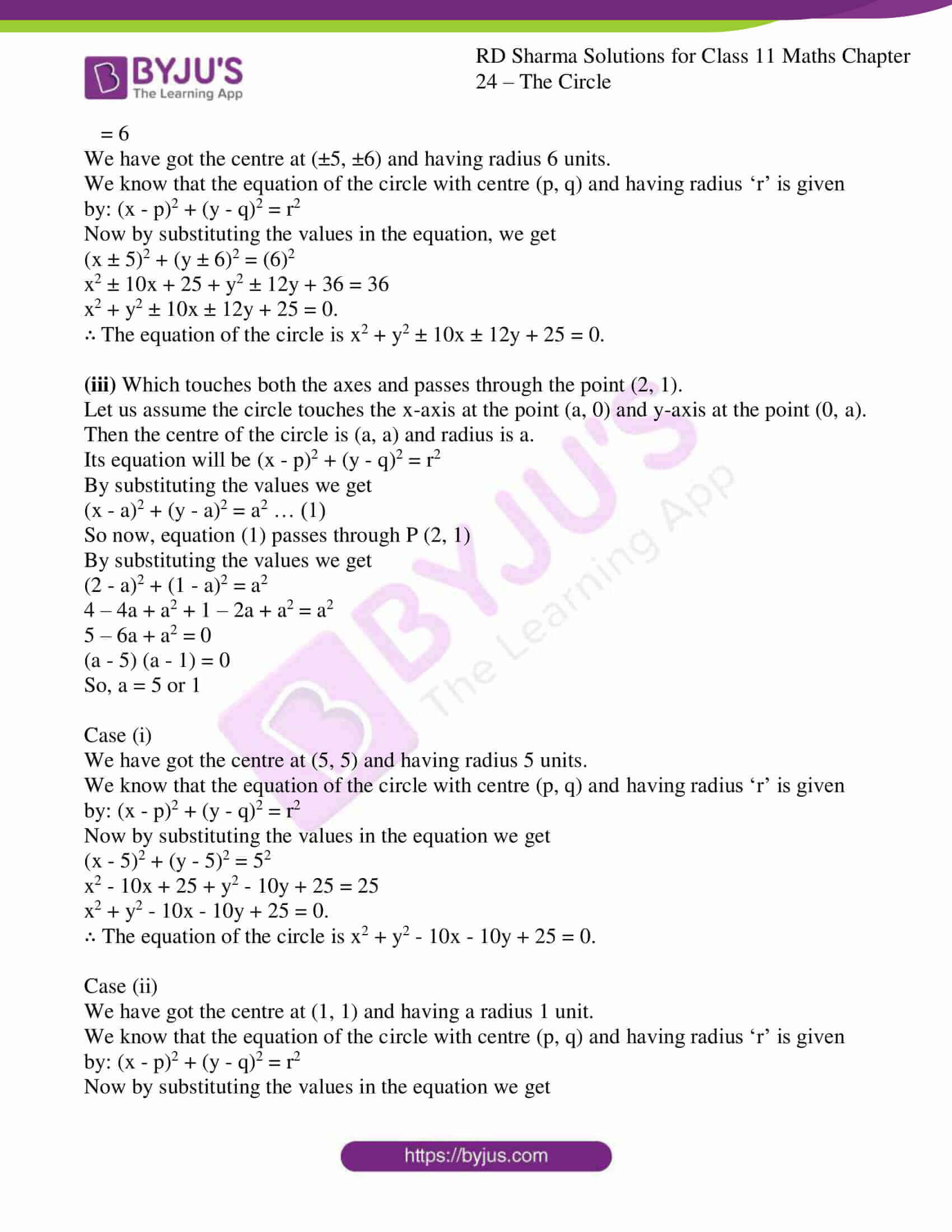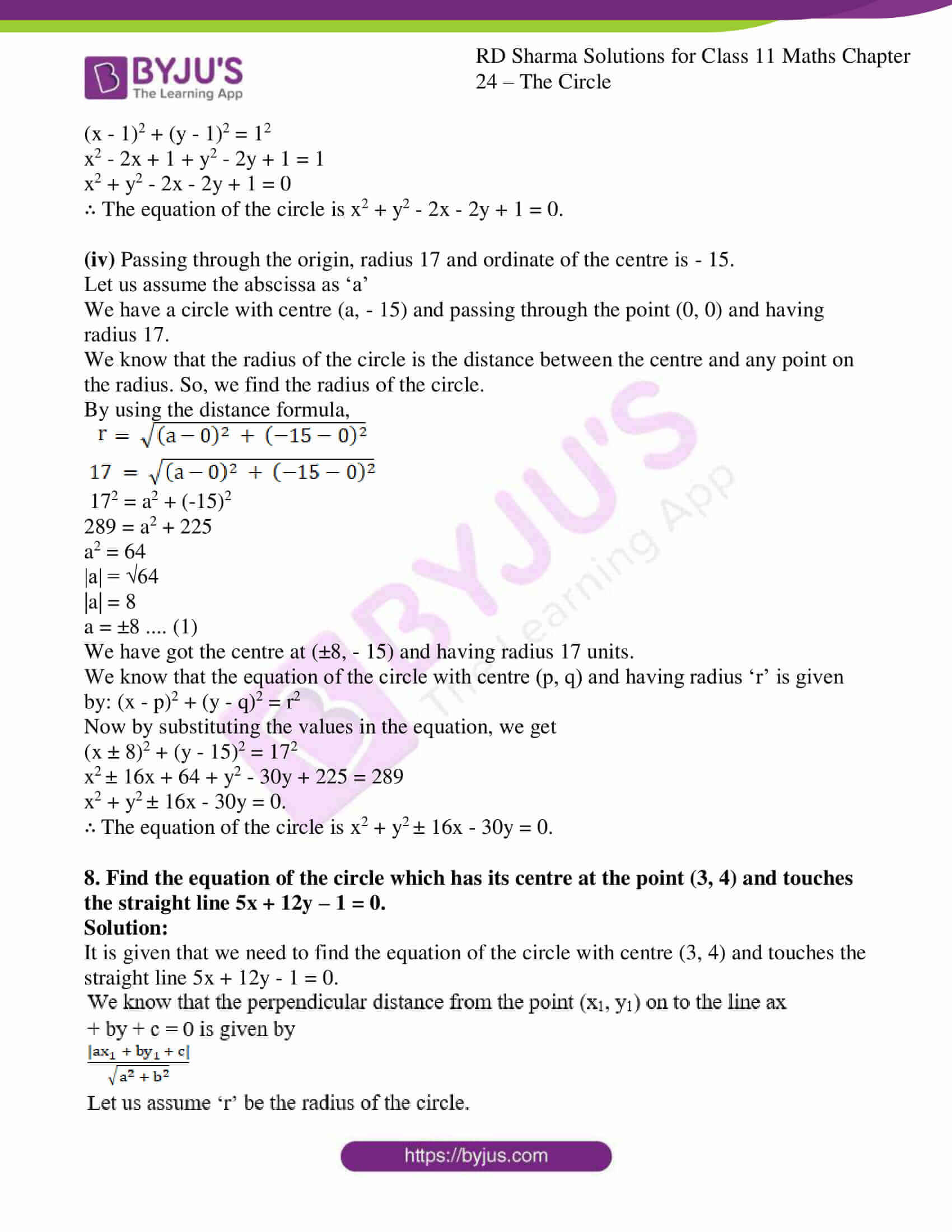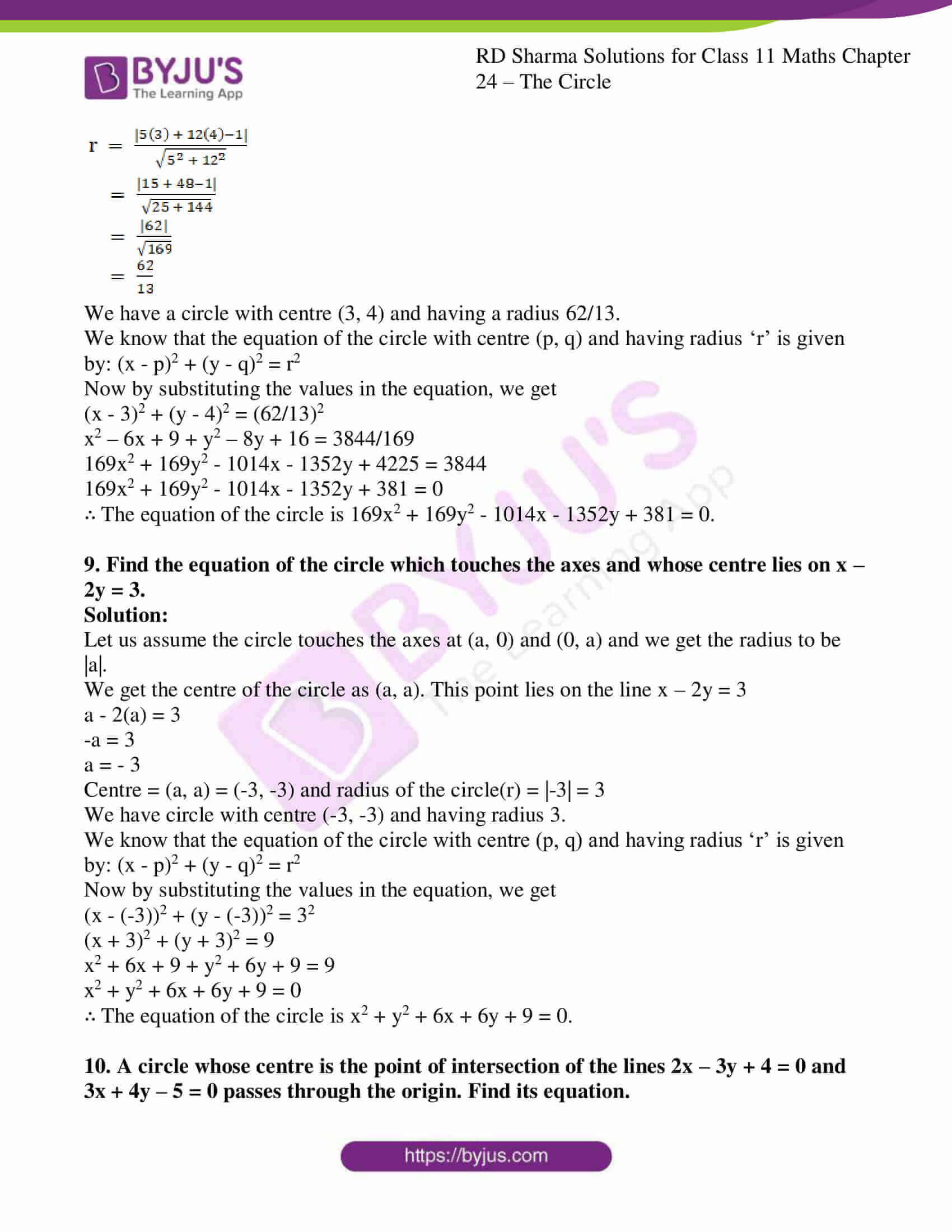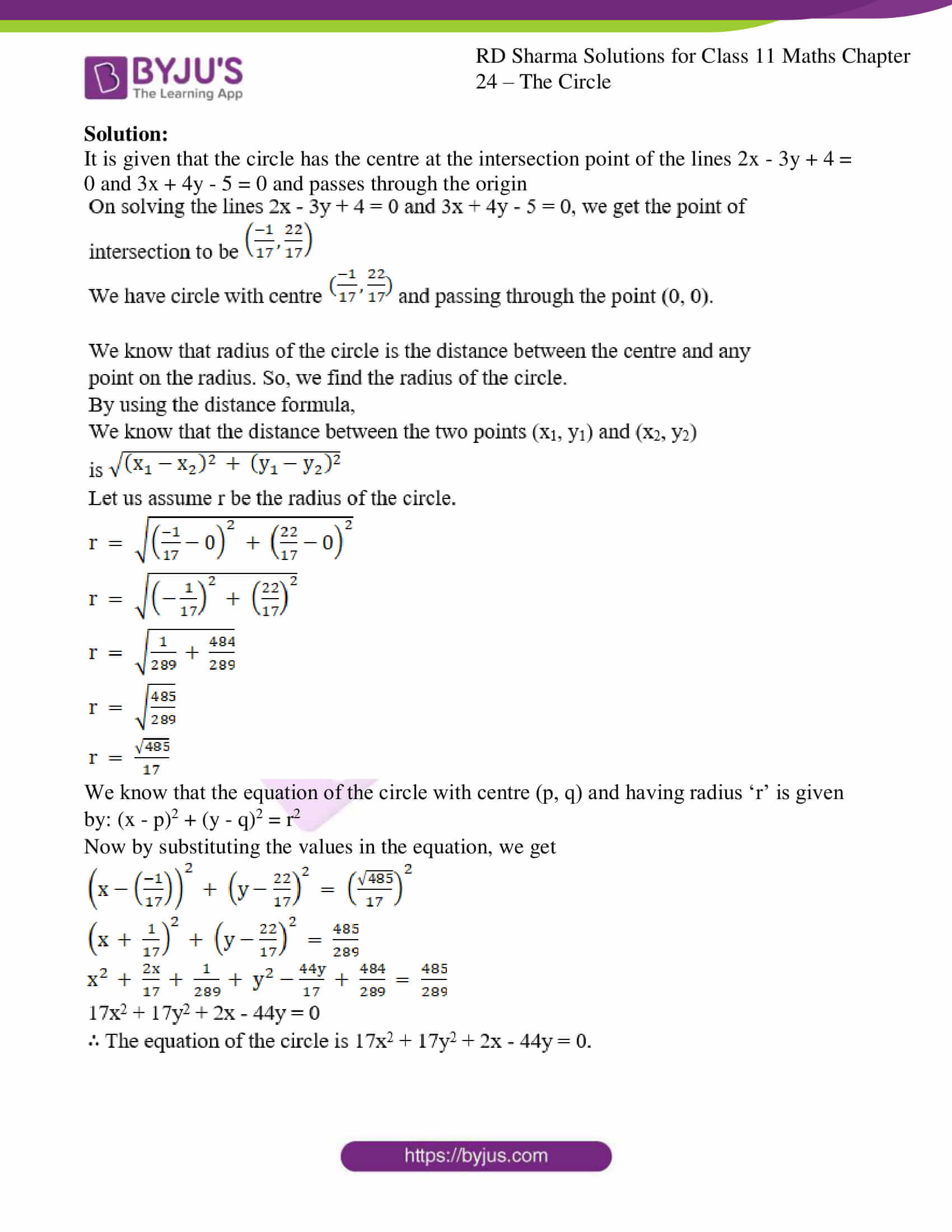### Access answers to RD Sharma Solutions for Class 11 Maths Exercise 24.1 Chapter 24 – The Circle

1. Find the equation of the circle with:
(i) Centre (-2, 3) and radius 4.

(ii) Centre (a, b) and radius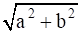.

(iii) Centre (0, – 1) and radius 1.

(iv) Centre (a cos α, a sin α) and radius a.

(v) Centre (a, a) and radius √2 a.

Solution:

(i) Centre (-2, 3) and radius 4.

Given:

The radius is 4 and the centre (-2, 3)

By using the formula,

The equation of the circle with centre (p, q) and radius ‘r’ is (x – p)2 + (y – q)2 = r2

Where, p = -2, q = 3, r = 4

Now by substituting the values in the above equation, we get

(x – p)2 + (y – q)2 = r2

(x – (-2))2 + (y – 3)2 = 42

(x + 2)2 + (y – 3)2 = 16

x2 + 4x + 4 + y2 – 6y + 9 = 16

x2 + y2 + 4x – 6y – 3 = 0

∴ The equation of the circle is x2 + y2 + 4x – 6y – 3 = 0

(ii) Centre (a, b) and radius.

Given:and the centre (a, b)

By using the formula,

The equation of the circle with centre (p, q) and radius ‘r’ is (x – p)2 + (y – q)2 = r2

Where, p = a, q = b, r =Now by substituting the values in the above equation, we get

(x – p)2 + (y – q)2 = r2

(x – a)2 + (y – b)2 =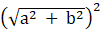x2 – 2ax + a2 + y2 – 2by + b2 = a2 + b2

x2 + y2 – 2ax – 2by = 0

∴ The equation of the circle is x2 + y2 – 2ax – 2by = 0

(iii) Centre (0, -1) and radius 1.

Given:

The radius is 1 and the centre (0, -1)

By using the formula,

The equation of the circle with centre (p, q) and radius ‘r’ is (x – p)2 + (y – q)2 = r2

Where, p = 0, q = -1, r = 1

Now by substituting the values in the above equation, we get

(x – p)2 + (y – q)2 = r2

(x – 0)2 + (y – (-1))2 = 12

(x – 0)2 + (y + 1)2 = 1

x2 + y2 + 2y + 1 = 1

x2 + y2 + 2y = 0

∴ The equation of the circle is x2 + y2 + 2y = 0.

(iv) Centre (a cos α, a sin α) and radius a.

Given:

The radius is ‘a’ and the centre (a cos α, a sin α)

By using the formula,

The equation of the circle with centre (p, q) and radius ‘r’ is (x – p)2 + (y – q)2 = r2

Where, p = a cos α, q = a sin α, r = a

Now by substituting the values in the above equation, we get

(x – p)2 + (y – q)2 = r2

(x – a cosα)2 + (y – a sinα)2 = a2

x2 – (2acosα)x + a2cos2α + y2 – (2asinα)y + a2sin2α = a2

We know that sin2θ + cos2θ = 1

So,

x2 – (2acosα)x + y2 – 2asinαy + a2 = a2

x2 + y2 – (2acosα)x – (2asinα)y = 0

∴ The equation of the circle is x2 + y2 – (2acosα) x – (2asinα) y = 0.

(v) Centre (a, a) and radius √2 a.

Given:

The radius is √2 a and the centre (a, a)

By using the formula,

The equation of the circle with centre (p, q) and radius ‘r’ is (x – p)2 + (y – q)2 = r2

Where, p = a, q = a, r = √2 a

Now by substituting the values in the above equation, we get

(x – p)2 + (y – q)2 = r2

(x – a)2 + (y – a)2 = (√2 a)2

x2 – 2ax + a2 + y2 – 2ay + a2 = 2a2

x2 + y2 – 2ax – 2ay = 0

∴ The equation of the circle is x2 + y2 – 2ax – 2ay = 0.

2. Find the centre and radius of each of the following circles:
(i) (x – 1)2 + y2 = 4

(ii) (x + 5)2 + (y + 1)2 = 9

(iii) x2 + y2 – 4x + 6y = 5

(iv) x2 + y2 – x + 2y – 3 = 0

Solution:

(i) (x – 1)2 + y2 = 4

Given:

The equation (x – 1)2 + y2 = 4

We need to find the centre and the radius.

By using the standard equation formula,

(x – a)2 + (y – b)2 = r2 …. (1)

Now let us convert given circle’s equation into the standard form.

(x – 1)2 + y2 = 4

(x – 1)2 + (y – 0)2 = 22 ….. (2)

By comparing equation (2) with (1), we get

Centre = (1, 0) and radius = 2

∴ The centre of the circle is (1, 0) and the radius is 2.

(ii) (x + 5)2 + (y + 1)2 = 9

Given:

The equation (x + 5)2 + (y + 1)2 = 9

We need to find the centre and the radius.

By using the standard equation formula,

(x – a)2 + (y – b)2 = r2 …. (1)

Now let us convert given circle’s equation into the standard form.

(x + 5)2 + (y + 1)2 = 9

(x – (-5))2 + (y – ( – 1))2 = 32 …. (2)

By comparing equation (2) with (1), we get

Centre = (-5, -1) and radius = 3

∴ The centre of the circle is (-5, -1) and the radius is 3.

(iii) x2 + y2 – 4x + 6y = 5

Given:

The equation x2 + y2 – 4x + 6y = 5

We need to find the centre and the radius.

By using the standard equation formula,

(x – a)2 + (y – b)2 = r2 …. (1)

Now let us convert given circle’s equation into the standard form.

x2 + y2 – 4x + 6y = 5

(x2 – 4x + 4) + (y2 + 6y + 9) = 5 + 4 + 9

(x – 2)2 + (y + 3)2 = 18

(x – 2)2 + (y – (-3))2 = (3√2)2 … (2)

By comparing equation (2) with (1), we get

Centre = (2, -3) and radius = 3√2

∴ The centre of the circle is (2, -3) and the radius is 3√2.

(iv) x2 + y2 – x + 2y – 3 = 0

Given:

The equation x2 + y2 – x + 2y – 3 = 0

We need to find the centre and the radius.

By using the standard equation formula,

(x – a)2 + (y – b)2 = r2 …. (1)

Now let us convert given circle’s equation into the standard form.

x2 + y2 – x + 2y – 3 = 0

(x2 – x + ¼) + (y2 + 2y + 1) – 3 – ¼ – 1 = 0

(x – ½)2 + (y + 1)2 = 17/4 …. (2)

By comparing equation (2) with (1), we get

Centre = (½, – 1) and radius = √17/2

∴ The centre of the circle is (½, -1) and the radius is √17/2.

3. Find the equation of the circle whose centre is (1, 2) and which passes through the point (4, 6).

Solution:

Given:

Centre is (1, 2) and which passes through the point (4, 6).

Where, p = 1, q = 2

We need to find the equation of the circle.

By using the formula,

(x – p)2 + (y – q)2 = r2

(x – 1)2 + (y – 2)2 = r2

It passes through the point (4, 6)

(4 – 1)2 + (6 – 2)2 = r2

32 + 42 = r2

9 + 16 = r2

25 = r2

r = √25

= 5

So r = 5 units

We know that the equation of the circle with centre (p, q) and having radius ‘r’ is given by: (x – p)2 + (y – q)2 = r2

By substitute the values in the above equation, we get

(x – 1)2 + (y – 2)2 = 52

x2 – 2x + 1 + y2 – 4y + 4 = 25

x2 + y2 – 2x – 4y – 20 = 0.

∴ The equation of the circle is x2 + y2 – 2x – 4y – 20 = 0.

4. Find the equation of the circle passing through the point of intersection of the lines x + 3y = 0 and 2x – 7y = 0 and whose centre is the point of intersection of the lines x + y + 1 = 0 and x – 2y + 4 = 0.

Solution:

Let us find the points of intersection of the lines.

On solving the lines x + 3y = 0 and 2x – 7y = 0, we get the point of intersection to be (0, 0)

On solving the lines x + y + 1 and x – 2y + 4 = 0, we get the point of intersection to be (-2, 1)

We have circle with centre (-2, 1) and passing through the point (0, 0).

We know that the radius of the circle is the distance between the centre and any point on the radius. So, we find the radius of the circle.

So, the equation is (x – p)2 + (y – q)2 = r2

Where, p = -2, q = 1

(x + 2)2 + (y – 1)2 = r2 …. (1)

Equation (1) passes through (0, 0)

So, (0 + 2)2 + (0 – 1)2 = r2

4 + 1 = a2

5 = r2

r = √5

We know that the equation of the circle with centre (p, q) and having radius ‘r’ is given by: (x – p)2 + (y – q)2 = r2

By substitute the values in the above equation, we get

(x – (-2))2 + (y – 1)2 = (√5)2

(x + 2)2 + (y – 1)2 = 5

x2 + 4x + 4 + y2 – 2y + 1 = 5

x2 + y2 + 4x – 2y = 0

∴ The equation of the circle is x2 + y2 + 4x – 2y = 0.

5. Find the equation of the circle whose centre lies on the positive direction of y – axis at a distance 6 from the origin and whose radius is 4.

Solution:

It is given that the centre lies on the positive y – axis at a distance of 6 from the origin, we get the centre (0, 6).

We have a circle with centre (0, 6) and having radius 4.

We know that the equation of the circle with centre (p, q) and having radius ‘r’ is given by: (x – p)2 + (y – q)2 = r2

Where, p = 0, q = 6, r = 4

Now by substituting the values in the equation, we get

(x – 0)2 + (y – 6)2 = 42

x2 + y2 – 12y + 36 = 16

x2 + y2 – 12y + 20 = 0.

∴ The equation of the circle is x2 + y2 – 12y + 20 = 0.

6. If the equations of two diameters of a circle are 2x + y = 6 and 3x + 2y = 4 and the radius is 10, find the equation of the circle.

Solution:

It is given that the circle has the radius 10 and has diameters 2x + y = 6 and 3x + 2y = 4.

We know that the centre is the intersection point of the diameters.

On solving the diameters, we get the centre to be (8, -10).

We have a circle with centre (8, -10) and having radius 10.

By using the formula,

We know that the equation of the circle with centre (p, q) and having radius ‘r’ is given by: (x – p)2 + (y – q)2 = r2

Where, p = 8, q = -10, r = 10

Now by substituting the values in the equation, we get

(x – 8)2 + (y – (-10))2 = 102

(x – 8)2 + (y + 10)2 = 100

x2 – 16x + 64 + y2 + 20y + 100 = 100

x2 + y2 – 16x + 20y + 64 = 0.

∴ The equation of the circle is x2 + y2 – 16x + 20y + 64 = 0.

7. Find the equation of the circle
(i) which touches both the axes at a distance of 6 units from the origin.

(ii) Which touches x – axis at a distance of 5 from the origin and radius 6 units.

(iii) Which touches both the axes and passes through the point (2, 1).

(iv) Passing through the origin, radius 17 and ordinate of the centre is – 15.

Solution:

(i) which touches both the axes at a distance of 6 units from the origin.

A circle touches the axes at the points (±6, 0) and (0, ±6).

So, a circle has a centre (±6, ±6) and passes through the point (0, 6).

We know that the radius of the circle is the distance between the centre and any point on the radius. So, we find the radius of the circle.

So, the equation is (x – p)2 + (y – q)2 = r2

Where, p = 6, q = 6

(x – 6)2 + (y – 6)2 = r2 …. (1)

Equation (1) passes through (0, 6)

So, (0 – 6)2 + (6 – 6)2 = r2

36 + 0 = r2

r = √36

= 6

We know that the equation of the circle with centre (p, q) and having radius ‘r’ is given by: (x – p)2 + (y – q)2 = r2

Now by substituting the values in the equation, we get

(x ± 6)2 + (y ± 6)2 = (6)2

x2 ± 12x + 36 + y2 ± 12y + 36 = 36

x2 + y2 ± 12x ± 12y + 36 = 0

∴ The equation of the circle is x2 + y2 ± 12x ± 12y + 36 = 0.

(ii) Which touches x – axis at a distance of 5 from the origin and radius 6 units.

A circle touches the x – axis at the points (±5, 0).

Let us assume the centre of the circle is (±5, a).

We have a circle with centre (5, a) and passing through the point (5, 0) and having radius 6.

We know that the radius of the circle is the distance between the centre and any point on the radius. So, we find the radius of the circle.

So, the equation is (x – p)2 + (y – q)2 = r2

Where, p = 5, q = a

(x – 5)2 + (y – a)2 = r2 …. (1)

Equation (1) passes through (5, 0)

So, (5 – 5)2 + (0 – 6)2 = r2

0 + 36 = r2

r = √36

= 6

We have got the centre at (±5, ±6) and having radius 6 units.

We know that the equation of the circle with centre (p, q) and having radius ‘r’ is given by: (x – p)2 + (y – q)2 = r2

Now by substituting the values in the equation, we get

(x ± 5)2 + (y ± 6)2 = (6)2

x2 ± 10x + 25 + y2 ± 12y + 36 = 36

x2 + y2 ± 10x ± 12y + 25 = 0.

∴ The equation of the circle is x2 + y2 ± 10x ± 12y + 25 = 0.

(iii) Which touches both the axes and passes through the point (2, 1).

Let us assume the circle touches the x-axis at the point (a, 0) and y-axis at the point (0, a).

Then the centre of the circle is (a, a) and radius is a.

Its equation will be (x – p)2 + (y – q)2 = r2

By substituting the values we get

(x – a)2 + (y – a)2 = a2 … (1)

So now, equation (1) passes through P (2, 1)

By substituting the values we get

(2 – a)2 + (1 – a)2 = a2

4 – 4a + a2 + 1 – 2a + a2 = a2

5 – 6a + a2 = 0

(a – 5) (a – 1) = 0

So, a = 5 or 1

Case (i)

We have got the centre at (5, 5) and having radius 5 units.

We know that the equation of the circle with centre (p, q) and having radius ‘r’ is given by: (x – p)2 + (y – q)2 = r2

Now by substituting the values in the equation we get

(x – 5)2 + (y – 5)2 = 52

x2 – 10x + 25 + y2 – 10y + 25 = 25

x2 + y2 – 10x – 10y + 25 = 0.

∴ The equation of the circle is x2 + y2 – 10x – 10y + 25 = 0.

Case (ii)

We have got the centre at (1, 1) and having a radius 1 unit.

We know that the equation of the circle with centre (p, q) and having radius ‘r’ is given by: (x – p)2 + (y – q)2 = r2

Now by substituting the values in the equation we get

(x – 1)2 + (y – 1)2 = 12

x2 – 2x + 1 + y2 – 2y + 1 = 1

x2 + y2 – 2x – 2y + 1 = 0

∴ The equation of the circle is x2 + y2 – 2x – 2y + 1 = 0.

(iv) Passing through the origin, radius 17 and ordinate of the centre is – 15.

Let us assume the abscissa as ‘a’

We have a circle with centre (a, – 15) and passing through the point (0, 0) and having radius 17.

We know that the radius of the circle is the distance between the centre and any point on the radius. So, we find the radius of the circle.

By using the distance formula,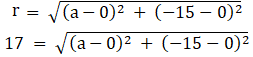172 = a2 + (-15)2

289 = a2 + 225

a2 = 64

|a| = √64

|a| = 8

a = ±8 …. (1)

We have got the centre at (±8, – 15) and having radius 17 units.

We know that the equation of the circle with centre (p, q) and having radius ‘r’ is given by: (x – p)2 + (y – q)2 = r2

Now by substituting the values in the equation, we get

(x ± 8)2 + (y – 15)2 = 172

x2 ± 16x + 64 + y2 – 30y + 225 = 289

x2 + y2 ± 16x – 30y = 0.

∴ The equation of the circle is x2 + y2 ± 16x – 30y = 0.

8. Find the equation of the circle which has its centre at the point (3, 4) and touches the straight line 5x + 12y – 1 = 0.

Solution:

It is given that we need to find the equation of the circle with centre (3, 4) and touches the straight line 5x + 12y – 1 = 0.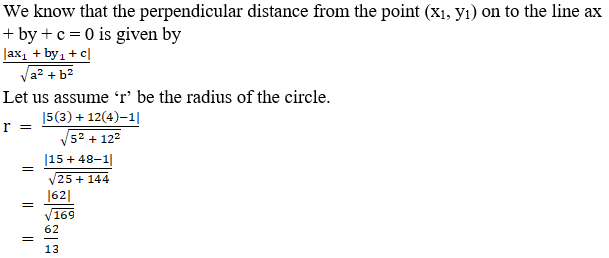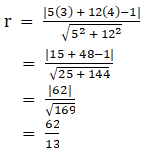We have a circle with centre (3, 4) and having a radius 62/13.

We know that the equation of the circle with centre (p, q) and having radius ‘r’ is given by: (x – p)2 + (y – q)2 = r2

Now by substituting the values in the equation, we get

(x – 3)2 + (y – 4)2 = (62/13)2

x2 – 6x + 9 + y2 – 8y + 16 = 3844/169

169x2 + 169y2 – 1014x – 1352y + 4225 = 3844

169x2 + 169y2 – 1014x – 1352y + 381 = 0

∴ The equation of the circle is 169x2 + 169y2 – 1014x – 1352y + 381 = 0.

9. Find the equation of the circle which touches the axes and whose centre lies on x – 2y = 3.

Solution:

Let us assume the circle touches the axes at (a, 0) and (0, a) and we get the radius to be |a|.

We get the centre of the circle as (a, a). This point lies on the line x – 2y = 3

a – 2(a) = 3

-a = 3

a = – 3

Centre = (a, a) = (-3, -3) and radius of the circle(r) = |-3| = 3

We have circle with centre (-3, -3) and having radius 3.

We know that the equation of the circle with centre (p, q) and having radius ‘r’ is given by: (x – p)2 + (y – q)2 = r2

Now by substituting the values in the equation, we get

(x – (-3))2 + (y – (-3))2 = 32

(x + 3)2 + (y + 3)2 = 9

x2 + 6x + 9 + y2 + 6y + 9 = 9

x2 + y2 + 6x + 6y + 9 = 0

∴ The equation of the circle is x2 + y2 + 6x + 6y + 9 = 0.

10. A circle whose centre is the point of intersection of the lines 2x – 3y + 4 = 0 and 3x + 4y – 5 = 0 passes through the origin. Find its equation.

Solution:

It is given that the circle has the centre at the intersection point of the lines 2x – 3y + 4 = 0 and 3x + 4y – 5 = 0 and passes through the origin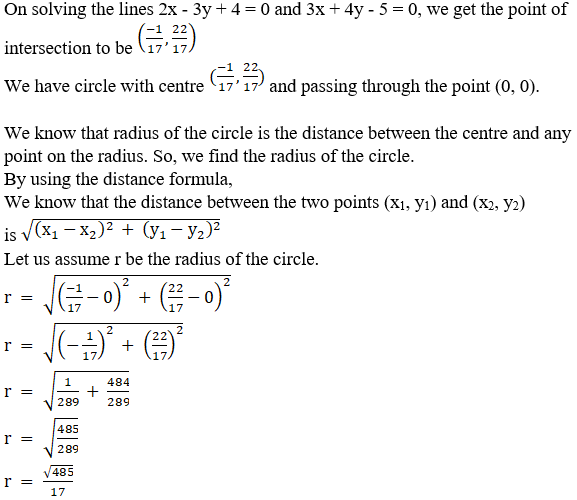We know that the equation of the circle with centre (p, q) and having radius ‘r’ is given by: (x – p)2 + (y – q)2 = r2

Now by substituting the values in the equation, we get